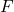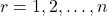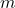# On generalized order statistics from exponential distribution

Letbe a non-negative random variable (r.v.) with cumulative distribution
function (cdf). Suppose, (),is a real number and, is the rth smallest generalized order statistic. Several distributional properties
of the generalized order statistics from exponential distribution are given.
Some characterizations of the exponential distribution based on the generalized
order statistics are presented.

Fulltext